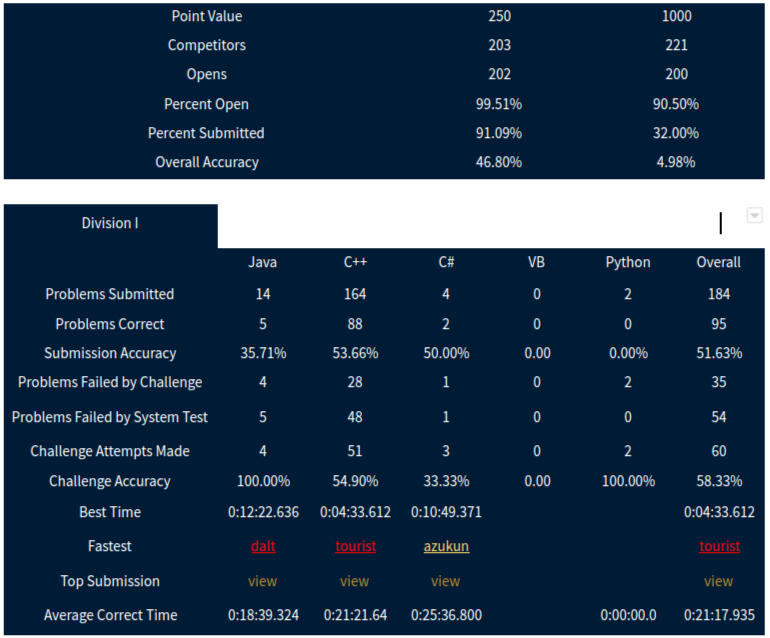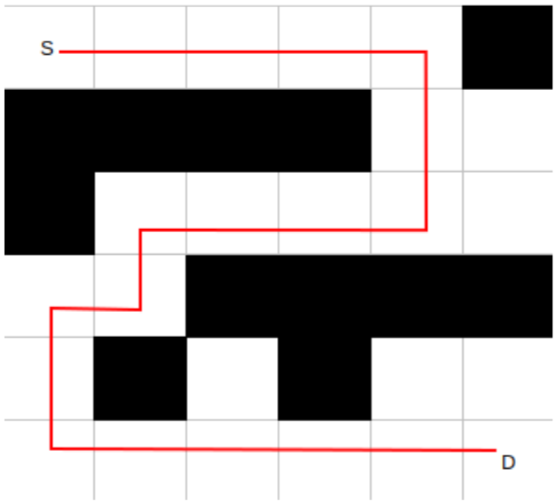AmirReza PoorAkhavana.poorakhavan

10min

# Problem of January '21

Hey!

For this problem post I sought a problem with a low success level in Div. 2, as I thought it would help more programmers, especially those beginners that want to challenge themselves using Div. 2 hard problems. So, I found FlightPlan.

Here are some of the problem stats from the contest:The problem includes a city with buildings. Each building has a height and we’re on top of the top-left corner building and want to reach to the opposite corner.

Once I read the problem, my memories revived back to a year ago, when we (I and my team) participated in ICPC Asia Tehran Regional Contest 2019 (my last onsite contest before Covid-19). Take a look at problem E in the contest. The problem The Big Surprise is harder than FlightPlan in some aspects; it has larger constraints and also initial and source positions are not fixed and they are given in the input. On the other hand, the cost of going down, up and sideways is not defined. I remember this problem because we were the only team that solved it in the contest. Serendipity to The Rebellion.

You can check the scoreboard here.

Let’s take a look at the solution which is almost the same for both the problems.

The first observation is that we can first go higher and higher till reaching Z = mh, then we move in X and Y direction (sideways) and at last go lower and lower to land on the destination.

If we can find mh somehow, the rest is easy. Run a BFS like a normal 2D problem. By a normal 2D problem I mean the following:

Given a 2D map with some obstacles in some cells, find the shortest path between some two cells. This can be solved easily using a simple BFS.For example, the distance between S and D is 18 in the above.

In the z = mh, we have a 2D problem where obstacles are the cells in which H is strictly more than mh.

After we find the shortest path we need to add the costs for getting up to mh and down from mh to the height of the destination.

Note that it’s possible that reaching the destination cell (r, c) will be impossible on some mh.

So how to find mh? Let’s try over all possible mhs. There are so many possible mhs - maybe one million?

No, observe again! Not all of them are important. The only important zs are those which are equal to some of elements of H.

Final step. Calculating complexity: there are r * c different values to be tested as mh, for every mh we need O(r * c) for BFS, so the final complexity is O(r^2 * c^2).

Take a quick look at tourist’s code:

``````1
2
3
4
5
6
7
8
9
10
11
12
13
14
15
16
17
18
19
20
21
22
23
24
25
26
27
28
29
30
31
32
33
34
35
36
37
38
39
40
41
42
43
44
45
46
47
48
49
50
51
52
53
54
55
56
57
``````const int di = {
-1,
0,
1,
0
};
const int dj = {
0,
-1,
0,
1
};

long long FlightPlan::fly(int h, int w, vector < int > H, int cup, int cdn, int clr) {
vector < vector < int >> a(h, vector < int > (w));
for (int i = 0; i < h; i++) {
for (int j = 0; j < w; j++) {
a[i][j] = H[i * w + j];
}
}
set < int > hs;
for (int i = 0; i < h; i++) {
for (int j = 0; j < w; j++) {
if (a[i][j] >= max(a, a[h - 1][w - 1])) {
hs.insert(a[i][j]);
}
}
}
long long ans = (long long) 1e18;
for (int up: hs) {
vector < vector < int >> dist(h, vector < int > (w, -1));
dist = 0;
vector < pair < int, int >> que;
que.emplace_back(0, 0);
for (int b = 0; b < (int) que.size(); b++) {
for (int dir = 0; dir < 4; dir++) {
int ni = que[b].first + di[dir];
int nj = que[b].second + dj[dir];
if (ni >= 0 && nj >= 0 && ni < h && nj < w) {
if (a[ni][nj] <= up && dist[ni][nj] == -1) {
que.emplace_back(ni, nj);
dist[ni][nj] = dist[que[b].first][que[b].second] + 1;
}
}
}
}
if (dist[h - 1][w - 1] == -1) {
continue;
}
long long cur = 0;
cur += (up - a) * 1 LL * cup;
cur += (up - a[h - 1][w - 1]) * 1 LL * cdn;
cur += dist[h - 1][w - 1] * 1 LL * clr;
ans = min(ans, cur);
}
return ans;
}
``````